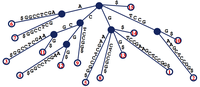# Finding the Longest Multiple Repeat solved by 577

Aug. 23, 2012, midnight by btjaden

Topics: Graph Algorithms

## Long Repeats

We saw in “Introduction to Pattern Matching” that a data structure commonly used to encode the relationships among a collection of strings was the trie, which is particularly useful when the strings represent a collection of patterns that we wish to match to a larger text.

The trie is helpful when processing multiple strings at once, but when we want to analyze a single string, we need something different.

In this problem, we will use a new data structure to handle the problem of finding long repeats in the genome. Recall from “Finding a Motif in DNA” that cataloguing these repeats is a problem of the utmost interest to molecular biologists, as a natural correlation exists between the frequency of a repeat and its influence on RNA transcription. Our aim is therefore to identify long repeats that occur more than some predetermined number of times.

## ProblemFigure 1. The suffix tree for s = GTCCGAAGCTCCGG. Note that the dollar sign has been appended to a substring of the tree to mark the end of s. Every path from the root to a leaf corresponds to a unique suffix of GTCCGAAGCTCCGG, and each leaf is labeled with the location in s of the suffix ending at that leaf.

A repeated substring of a string $s$ of length $n$ is simply a substring that appears in more than one location of $s$; more specifically, a k-fold substring appears in at least k distinct locations.

The suffix tree of $s$, denoted $T(s)$, is defined as follows:

• $T(s)$ is a rooted tree having exactly $n$ leaves.
• Every edge of $T(s)$ is labeled with a substring of $s^*$, where $s^*$ is the string formed by adding a placeholder symbol $ to the end of $s$. • Every internal node of $T(s)$other than the root has at least two children; i.e., it has degree at least 3. • The substring labels for the edges leading from a node to its children must begin with different symbols. • By concatenating the substrings along edges, each path from the root to a leaf corresponds to a unique suffix of $s^*$. See Figure 1 for an example of a suffix tree. Given: A DNA string $s$(of length at most 20 kbp) with $ appended, a positive integer $k$, and a list of edges defining the suffix tree of $s$. Each edge is represented by four components:

1. the label of its parent node in $T(s)$;
2. the label of its child node in $T(s)$;
3. the location of the substring $t$ of $s^{*}$ assigned to the edge; and
4. the length of $t$.

Return: The longest substring of $s$ that occurs at least $k$ times in $s$. (If multiple solutions exist, you may return any single solution.)